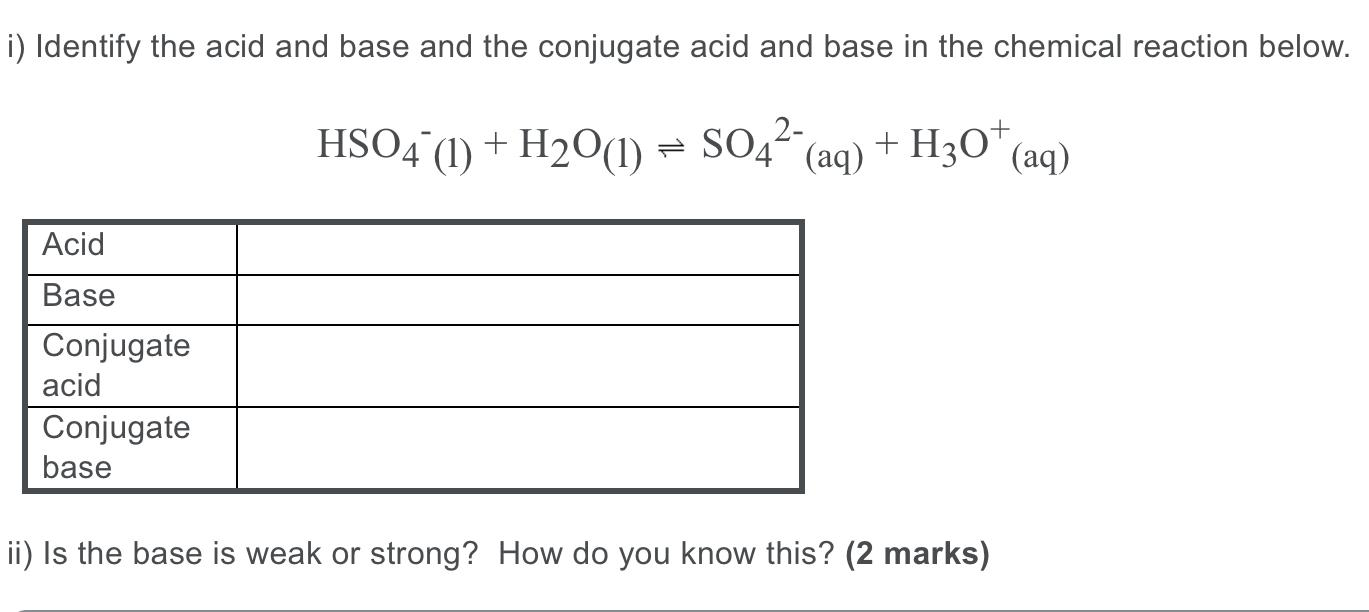# Question Solved1 Answeri) Identify the acid and base and the conjugate acid and base in the chemical reaction below. HS04 (1) + H2O(1) = 5042- + SO4 (aq) (aq) + H30+ (aq) Acid Base Conjugate acid Conjugate base ii) Is the base is weak or strong? How do you know this? (2 marks)Transcribed Image Text: i) Identify the acid and base and the conjugate acid and base in the chemical reaction below. HS04 (1) + H2O(1) = 5042- + SO4 (aq) (aq) + H30+ (aq) Acid Base Conjugate acid Conjugate base ii) Is the base is weak or strong? How do you know this? (2 marks)
More
Transcribed Image Text: i) Identify the acid and base and the conjugate acid and base in the chemical reaction below. HS04 (1) + H2O(1) = 5042- + SO4 (aq) (aq) + H30+ (aq) Acid Base Conjugate acid Conjugate base ii) Is the base is weak or strong? How do you know this? (2 marks)